GeeksforGeeks App
Open AppBrowser
Continue

# Create a Random Password Generator using Python

Passwords are a means by which a user proves that they are authorized to use a device. It is important that passwords must be long and complex. It should contain at least more than ten characters with a combination of characters such as percent (%), commas(,), and parentheses, as well as lower-case and upper-case alphabets and numbers. Here we will create a random password using Python code.

Example of a strong password : &gj5hj&*178a1

## Modules needed

string – For accessing string constants. The ones we would need are –

• string.ascii_letters:  ASCII is a system that is used to represent characters digitally, every ASCII character has its own unique code. string.ascii_letters is a string constant which contains all the letters in ASCII ranging from A to Z and a to z. Its value is non-locale dependent and it is just a concatenation of ascii_uppercase and ascii_lowercase. Thus it provides us the whole letter set as a string that can be used as desired.
• string.digits This is a pre-initialized string that contains all the digits in the Arabic numeral system i.e. 0, 1, 2, 3, 4, 5, 6, 7, 8, 9. It should be kept in mind that even though these are digits, the type is still a string constant, and all digits are concatenated like this – “0123456789”. If we want to access specific numbers then we can do so using slicing.
• string.punctuation: Apart from letters and digits, python also provides us all the special characters in a pre-initialized string constant. These include various kinds of braces, logical operators, comparison operators, arithmetical operators as well as punctuation marks like commas, inverted commas, periods, exclamations marks, and question marks. The whole string is – !”#\$%&'()*+, -./:;<=>?@[\]^_`{|}~

random – The python random module helps a user to generate pseudo-random numbers. Inside the module, there are various functions that just depend on the function “random()”. This function generates a random float uniformly in the semi-open range [0.0, 1.0) i.e. it generates a decimal number greater than or equal to 0 and strictly less than one. Other functions use this number in their own ways. These functions can be used for bytes, integers, and sequences. for our task, we are interested in sequences. There are functions random. choices that take in a sequence as its argument and return a random element from that sequence.

### Code Implementation:

First, take the length of the password as input. Then we can display a prompt about the possible list of characters that a user wants to include in the password –

• For including letters in the character set append string.ascii_letters in the character list.
• For including letters in the character set append string.digits in the character list.
• For including letters in character set append string.punctuation in the character list.

Run a for loop till the length of the password and in each iteration choose a random character using random.choice() from characterList. Finally, print the password.

## Python3

 `import` `string``import` `random` `# Getting password length``length ``=` `int``(``input``(``"Enter password length: "``))` `print``(``'''Choose character set for password from these :``         ``1. Digits``         ``2. Letters``         ``3. Special characters``         ``4. Exit'''``)` `characterList ``=` `""` `# Getting character set for password``while``(``True``):``    ``choice ``=` `int``(``input``(``"Pick a number "``))``    ``if``(choice ``=``=` `1``):``        ` `        ``# Adding letters to possible characters``        ``characterList ``+``=` `string.ascii_letters``    ``elif``(choice ``=``=` `2``):``        ` `        ``# Adding digits to possible characters``        ``characterList ``+``=` `string.digits``    ``elif``(choice ``=``=` `3``):``        ` `        ``# Adding special characters to possible``        ``# characters``        ``characterList ``+``=` `string.punctuation``    ``elif``(choice ``=``=` `4``):``        ``break``    ``else``:``        ``print``(``"Please pick a valid option!"``)` `password ``=` `[]` `for` `i ``in` `range``(length):``  ` `    ``# Picking a random character from our``    ``# character list``    ``randomchar ``=` `random.choice(characterList)``    ` `    ``# appending a random character to password``    ``password.append(randomchar)` `# printing password as a string``print``(``"The random password is "` `+` `"".join(password))`

Output:

Input 1: Taking only letters in the character set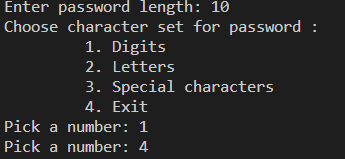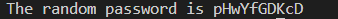Output

Input 1: Taking letters and numbers both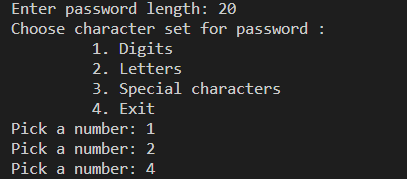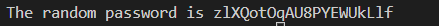Output

Input 3: Taking letters numbers as well as special characters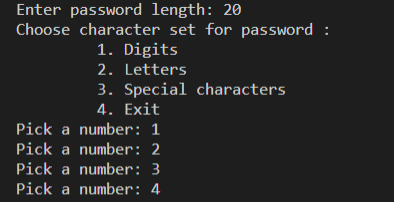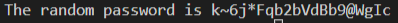Output

Implementation

## Python3

 `# import modules``import` `string``import` `random`  `# store all characters in lists``s1 ``=` `list``(string.ascii_lowercase)``s2 ``=` `list``(string.ascii_uppercase)``s3 ``=` `list``(string.digits)``s4 ``=` `list``(string.punctuation)`  `# Ask user about the number of characters``user_input ``=` `input``(``"How many characters do you want in your password? "``)`  `# check this input is it number? is it more than 8?``while` `True``:` `    ``try``:` `        ``characters_number ``=` `int``(user_input)` `        ``if` `characters_number < ``8``:` `            ``print``(``"Your number should be at least 8."``)` `            ``user_input ``=` `input``(``"Please, Enter your number again: "``)` `        ``else``:` `            ``break` `    ``except``:` `        ``print``(``"Please, Enter numbers only."``)` `        ``user_input ``=` `input``(``"How many characters do you want in your password? "``)`  `# shuffle all lists``random.shuffle(s1)``random.shuffle(s2)``random.shuffle(s3)``random.shuffle(s4)`  `# calculate 30% & 20% of number of characters``part1 ``=` `round``(characters_number ``*` `(``30``/``100``))``part2 ``=` `round``(characters_number ``*` `(``20``/``100``))`  `# generation of the password (60% letters and 40% digits & punctuations)``result ``=` `[]` `for` `x ``in` `range``(part1):` `    ``result.append(s1[x])``    ``result.append(s2[x])` `for` `x ``in` `range``(part2):` `    ``result.append(s3[x])``    ``result.append(s4[x])`  `# shuffle result``random.shuffle(result)`  `# join result``password ``=` `"".join(result)``print``(``"Strong Password: "``, password)`

Output: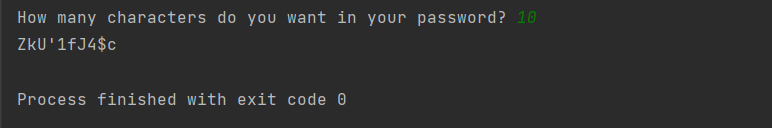Output

My Personal Notes arrow_drop_up